Published

# Splish Splash - I Was Taking A Bath

A way to provide safe and reliable bath water temperature readings to bathe your child or pet in.

IntermediateShowcase (no instructions)67## Things used in this project

### Hardware components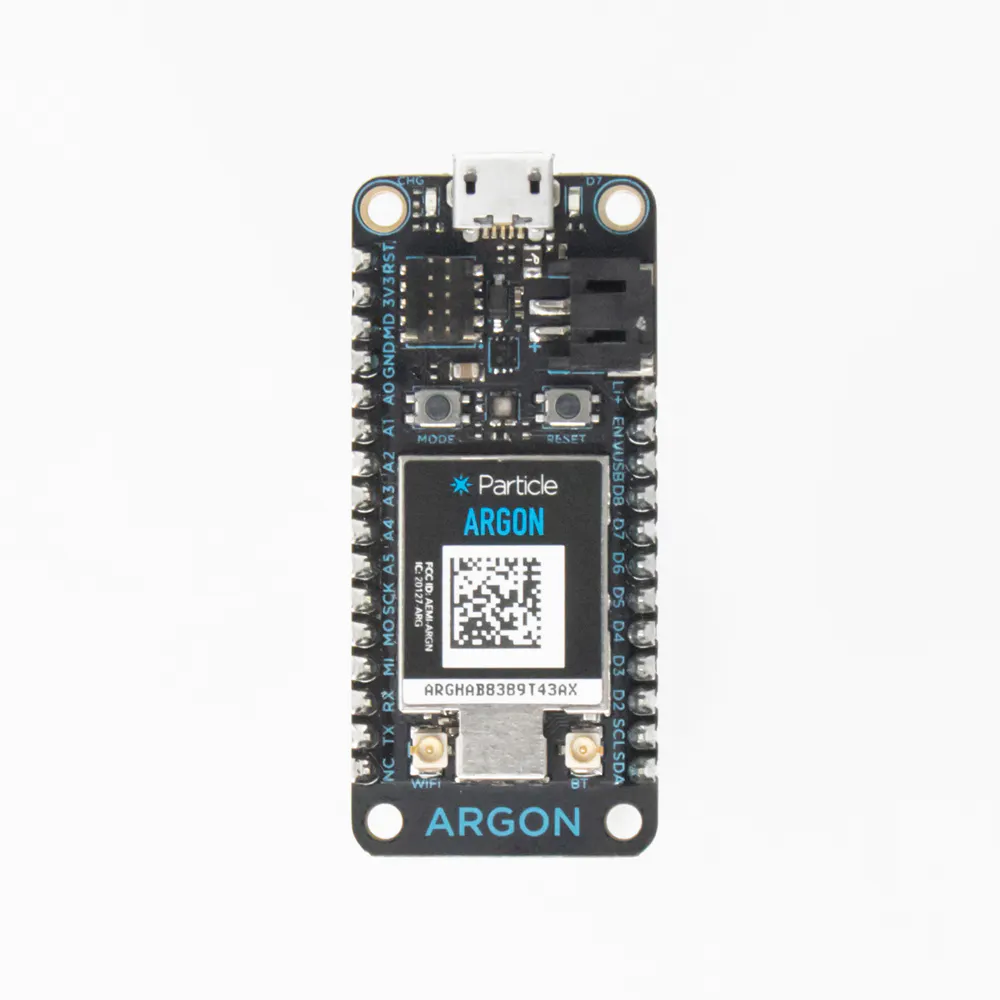Particle Argon
×2
×1Resistor 10k ohm
×1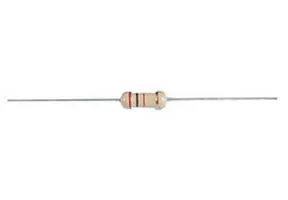Through Hole Resistor, 47 ohm
×3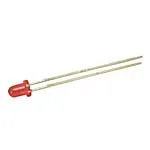3 mm LED: Red
×13 mm LED: Green
×1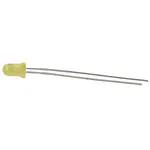3 mm LED: Yellow
×1Jumper wires (generic)
×1

### Software apps and online servicesParticle Build Web IDEThingSpeak API

## Schematics

### Temperature Sensor Circuit Diagram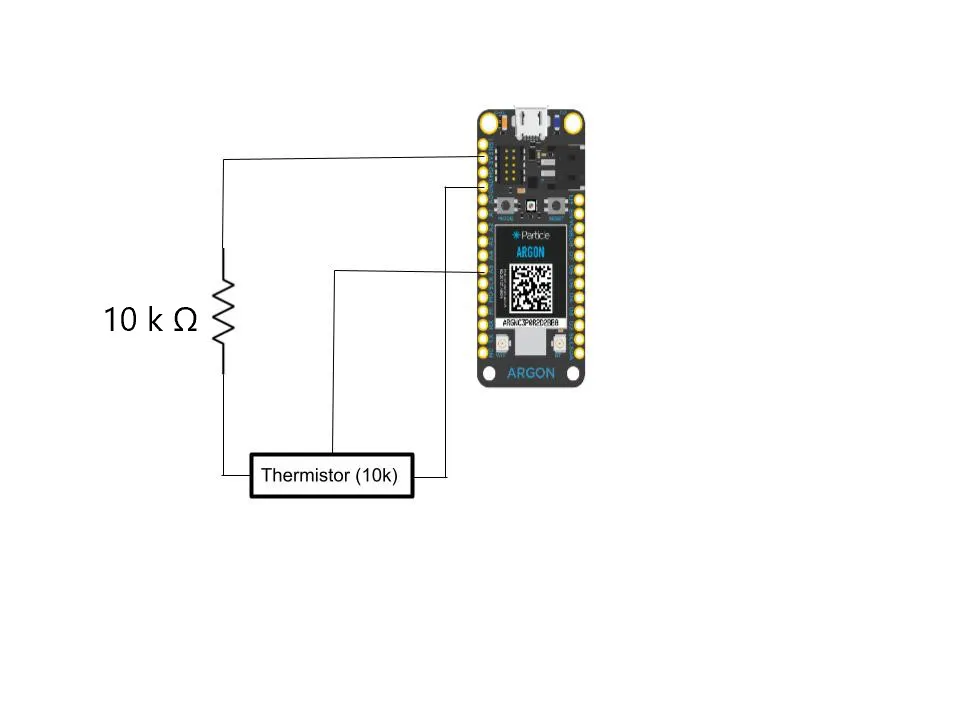### LED Sensor Circuit Diagram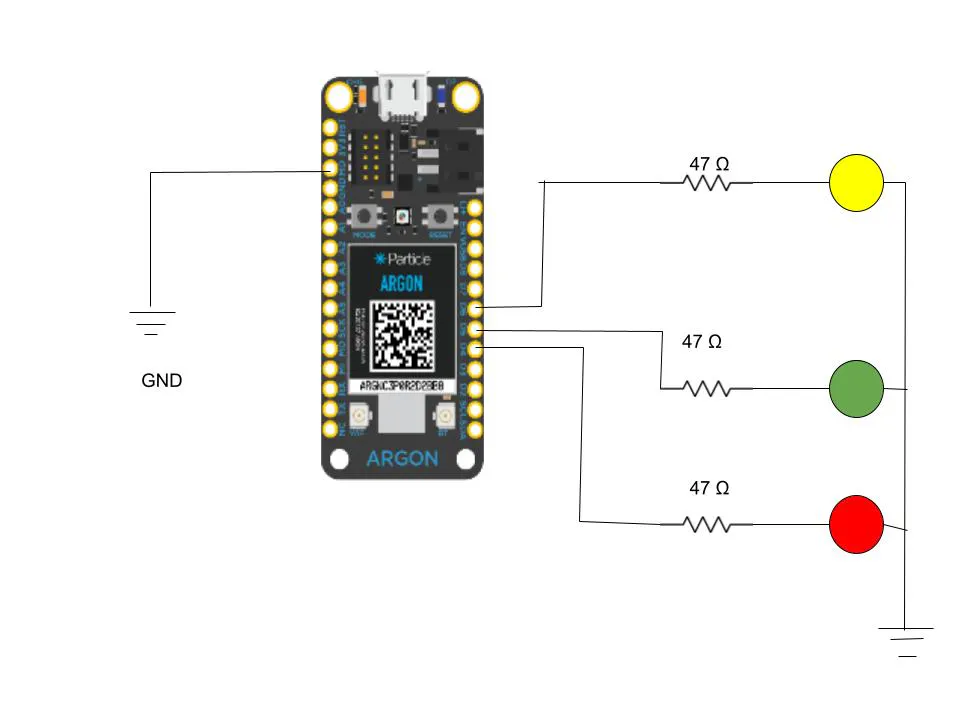## Code

### LED Sensor Code

C/C++
```float V=1;
int led1;
void setup() {
pinMode(D6, OUTPUT);
pinMode(D5, OUTPUT);
pinMode(D4, OUTPUT);
Particle.subscribe ("Temperature_Sensor", Light);
}
// Turns on the lights depending on the temperature range
void Light(const char *event, String data) {
led1=data.toInt();
if (led1<=40){
digitalWrite(D6, HIGH);
digitalWrite(D5, LOW);
digitalWrite(D4, LOW);
}
else if (led1>=40 && led1<= 50){
digitalWrite(D5, HIGH);
digitalWrite(D4, LOW);
digitalWrite(D6, LOW);

}
else if (led1 >= 50){
digitalWrite(D4, HIGH);
digitalWrite(D5, LOW);
digitalWrite(D6, LOW);
}
else {
digitalWrite(D6, LOW);
digitalWrite(D5, LOW);
digitalWrite(D4, LOW);
}
String connect = String(V);
Particle.publish("LED_Sensor");
```

### Temperature Code

C/C++
```#include <math.h>

int ThermistorPin = A5;
int Vo;
double R1 = 9970;
double logR, R2, T, Tc;
double c1 = 0.001129148, c2 = 0.000234125, c3= 0.0000000876741;

void setup() {
pinMode(D7, OUTPUT);
Particle.subscribe ("LED_Sensor", Lightaccr);
pinMode (ThermistorPin, INPUT);
}

void loop() {
// Gets data from the Thermistor and converts it into usable temperature data
R2 = R1/(4096.0/((double)Vo-1));
logR = log(R2);
T = (1.0 / (c1 + (c2 * logR) + c3 *( logR * logR * logR)));
Tc = T - 273.15;
// Sends the data to the other argon
Particle.publish("Temperature_Sensor", String(Tc));
//Sends the data to the live graph
Particle.publish("temp", String(Tc), PRIVATE);
// Wait 15 seconds
delay(15000);
}
// Acknowledgement that the data was received by the other Argon
void Lightaccr(const char *eventName, const char *data)
{
digitalWrite(D7, HIGH);
}
```

## Credits

### Katelyn Fennell

1 project • 0 followers